Next: Steady flows of incompressible Up: Fluid Mechanics: Preliminaries Previous: Streamlines and streamtubes

# Circulation, vorticity, and vortices

Fluids will invariably swirl around, and it is often useful to characterize the amount of swirling. This is done by introducing the circulation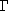, defined as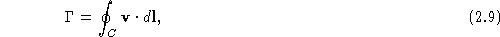with the line integral taken is around a closed circuit C in a counter-clockwise sense. By using Stokes' theorem we may write this as a surface integral,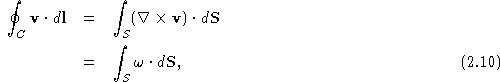where the vorticityof the fluid is defined as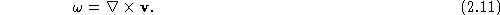If the vorticity of the fluid is zero, we say that the fluid is irrotational :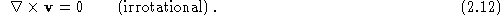We've already mentioned that there are some useful analogies between magnetostatics and incompressible fluid flow. I've collected these together in Table 2.1 below. To transform the magnetostatics expressions into those for fluid flow, we make the substitutions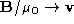,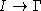, and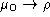(where hereis the mass density of the fluid). If a fluid is both incompressible and irrotational, then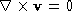and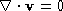, which are the same as the equations of electrostatics and magnetostatics in free space (i.e., in the absence of charges and currents).

 Magnetostatics Incompressible fluid flow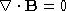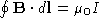(Ampére's Law)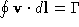(circulation)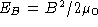(energy density of the magnetic field)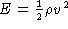(kinetic energy density of a fluid)

As an example of fluid flow with vorticity, we can consider a single vortex , which would be produced when draining a bathtub, and which is illustrated in Fig. 2.5.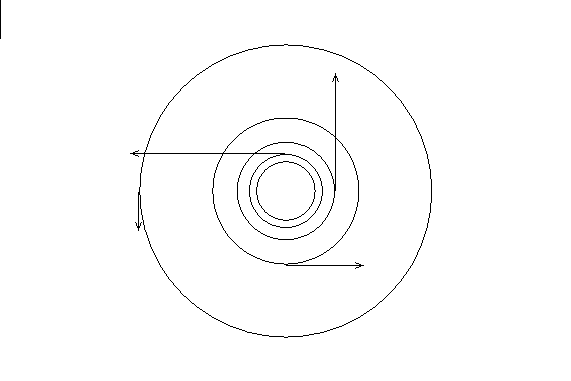Figure 2.5: Velocity field of a vortex. Flow lines are drawn for equal increments of the speed, as shown by arrows. Click here to see animations.

In cylindrical coordinates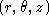there is only one component of the velocity field,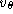. In calculating the circulation, the line element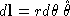, so that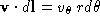. If the circulation is independent of the integration path, then we must have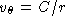, with C a constant. The circulation is then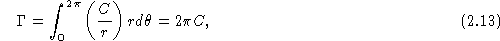so that. Therefore, the velocity field of a vortex is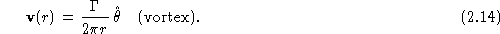By making the substitutions suggested above, we can use this result to find the magnetic field produced by a wire carrying a current I: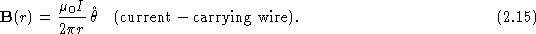Next: Steady flows of incompressible Up: Fluid Mechanics: Preliminaries Previous: Streamlines and streamtubes

Vittorio Celli
Wed Sep 10 01:02:02 EDT 1997